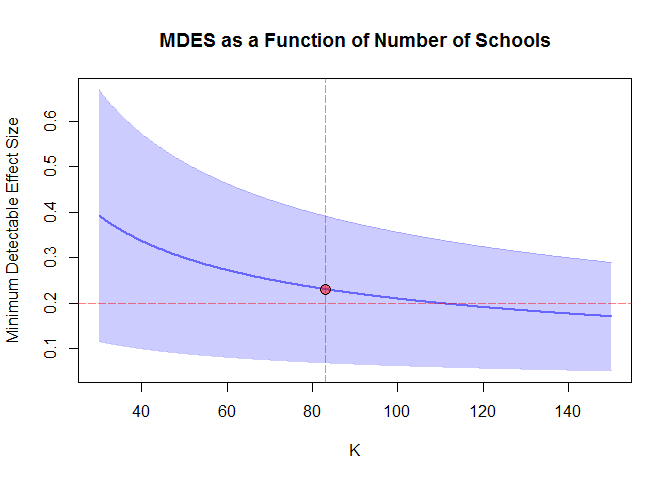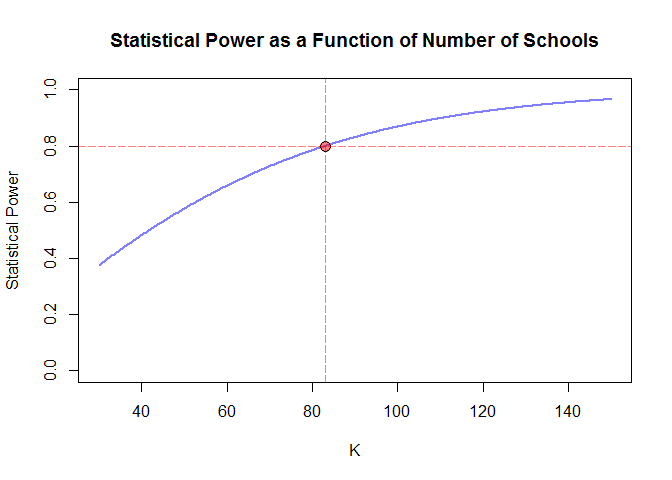# Planning a Three-Level Cluster Randomized Trial Sensitive to Detect Main Treatment Effect

#### 2019-03-09

To install and load PowerUpR:

install.packages("PowerUpR")
library(PowerUpR)

Institue of Education Science (IES) reported that evidence on effectiveness of Saxon Math curriclum on secondary school student’s algebra subject is lacking and further research is needed (U.S. Department of Education, 2016). To evaluate the effectiveness Saxon Math curriculum, assume a three-level cluster-randomized trial is considered where schools are randomly assigned to treatment (Saxon Math curriclum) and control conditions. In the grant proposal number of schools, clasrooms, and students needs to be justified. Due to nesting of students within classrooms and nesting of classrooms within schools, the treatment effect ($$\delta$$) can be estimated via the following three-level hierarchical linear model (Raudenbush & Bryk, 2002)

$\begin{eqnarray} L1: Y_{ijk} &=& \beta_{0jk} + \beta_{1jk}X + r_{ijk}, \quad r_{ijk} \thicksim N(0,\sigma_{|X}^2) \newline L2: \beta_{0jk} &=& \gamma_{00k} + \gamma_{01k}W + \mu_{0jk}, \quad \mu_{0jk} \thicksim N(0,\tau_{2|W}^2) \newline \beta_{1jk} &=& \gamma_{10k} \newline L3: \gamma_{00k} &=& \xi_{001} + \delta T_k + \xi_{002}V + \varsigma_{00k}, \quad \varsigma_{00k} \thicksim N(0,\tau_{3|V}^2) \newline \gamma_{01k} &=& \xi_{010} \newline \gamma_{10k} &=& \xi_{100} \end{eqnarray}$

where, $$Y$$: Student algebra posttest score. $$X$$: Student algebra pretest score. $$W$$: Classroom mean algebra pretest score. $$V$$: School mean algebra pretest score. $$T$$: Treatment status. Consider the following hypothetical values for design parameters

Proportion of variance in algebra posttest

• at the school level ($$\tau_3^2$$): $$.15$$
• at the classroom level ($$\tau_2^2$$): $$.05$$
• at the student level ($$\sigma^2$$): $$.65$$
• explained by school mean algebra pretest: ($$R_3^2 = 1 - \frac{\tau_{3|V}^2}{\tau_{3}^2}$$): $$.45$$
• explained by classroom mean algebra pretest: ($$R_2^2 = 1 - \frac{\tau_{2|W}^2}{\tau_{2}^2}$$): $$.50$$
• explained by student algebra pretest: ($$R_1^2 = 1 - \frac{\sigma_{|X}^2}{\sigma^2}$$): $$.55$$

Then, intraclass correlation coefficients are

• $$\rho_2 = \tau_2^2 / (\sigma^2 + \tau_2^2 + \tau_3^2) = .05 /(.65 + .15 + .05) = 0.059$$
• $$\rho_3 = \tau_3^2 / (\sigma^2 + \tau_2^2 + \tau_3^2) = .15 /(.65 + .15 + .05) = 0.176$$

Further assumptions

• Alpha level ($$\alpha$$): $$.05$$
• Number of schools ($$K$$): $$83$$
• Proportion of schools in treatment condition ($$p$$): $$.40$$
• Average number of classrooms per school ($$J$$): $$2$$
• Average number of students per classroom ($$n$$) : $$10$$
• Statistical power ($$1-\beta$$): $$.80$$

Given design parameters and further assumptions, what is the minimum detectable effect size?

mdes <- mdes.cra3r3(power=.80, rho2=.06, rho3=.18,
g3=1, r21=.55, r22=.50, r23=.45,
p=.40, n=10, J=2, K=83)
##
## Minimum detectable effect size:
## ---------------------------------------
## MDES is 0.23 95% CI [0.069,0.392] with 80% power
## ---------------------------------------
## Degrees of freedom: 80
## Standardized standard error: 0.081
## Type I error rate: 0.05
## Type II error rate: 0.2
## Two-tailed test: TRUE
plot(mdes, ypar = "mdes", xlim = c(30, 150),
main = "MDES as a Function of Number of Schools",
locate = TRUE)Given design parameters and further assumptions, what is the statistical power?

power <- power.cra3r3(es=.23, rho2=.06, rho3=.18,
g3=1, r21=.55, r22=.50, r23=.45,
p=.40, n=10, J=2, K=83)
##
## Statistical power:
## ---------------------------------------
##  80% power
## ---------------------------------------
## Degrees of freedom: 80
## Standardized standard error: 0.081
## Type I error rate: 0.05
## Type II error rate: 0.2
## Two-tailed test: TRUE
plot(power, ypar = "power", xlim = c(30, 150),
main = "Statistical Power as a Function of Number of Schools",
locate = TRUE)## Relevant Citations

Dong, N., & Maynard, R. A. (2013a). PowerUp!: A Tool for Calculating Minimum Detectable Effect Sizes and Minimum Required Sample Sizes for Experimental and Quasi-Experimental Design Studies, Journal of Research on Educational Effectiveness, 6(1), 24-6.

Dong, N., & Maynard, R. A. (2013b). PowerUp!: A tool for calculating minimum detectable effect sizes and minimum required sample sizes for experimental and quasi-experimental design studies. [Software]. Accessed from http://www.causalevaluation.org/.

U.S. Department of Education, Institute of Education Sciences, What Works Clearinghouse. (2016, May). Secondary Mathematics intervention report: Saxon Math. Retrieved from https://ies.ed.gov/ncee/wwc/Docs/InterventionReports/wwc_saxon_052416.pdf